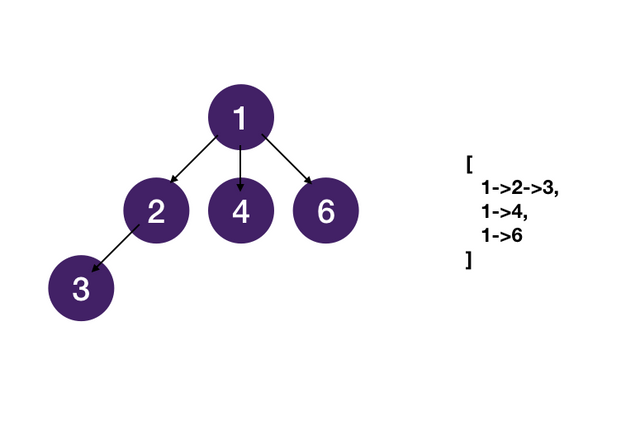# DFS with States

Prereq: DFS on Tree

Let's reinforce our understanding of state with one more example.

## Ternary Tree Paths

Given a ternary tree (each node of the tree has at most three children), find all root-to-leaf paths.## Try it yourself

We use `path` to keep track of the nodes we have visited to reach the current node and use it to construct our solution when we reach leaf nodes.

``````def ternary_tree_paths(root):
# dfs helper function
def dfs(root, path, res):
# exit condition, reached leaf node, append paths to results
if all(c is None for c in root.children):
res.append('->'.join(path) + '->' + root.val)
return

# dfs on each non-null child
for child in root.children:
if child is not None:
dfs(child, path + [root.val], res)

res = []
if root: dfs(root, [], res)
return res

``````

## Saving Space

In the recursive call in the previous solution, we create a new list each time we recurse with `path + [root.val]`. This is not space-efficient because creating a new list requires allocating new space in memory and copy over each element. A more efficient way is to use a single list and append/pop following the call stack.

 `Expand 5 lines ...` `6` `6` `7` `7` `` for child in root.children:`` `8` `8` `` if child is not None:`` `9` `-` `` dfs(child, path + [root.val], res)`` `9` `+` `` path.append(root.val)`` `10` `+` `` dfs(child, path, res)`` `11` `+` `` path.pop()`` `12` `+` `10` `13` `` res = []`` `11` `14` `` if root: dfs(root, [], res)`` `12` `15` `` return res``